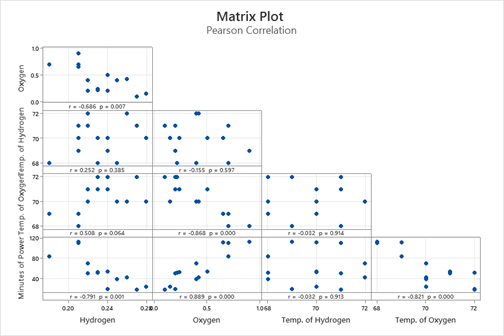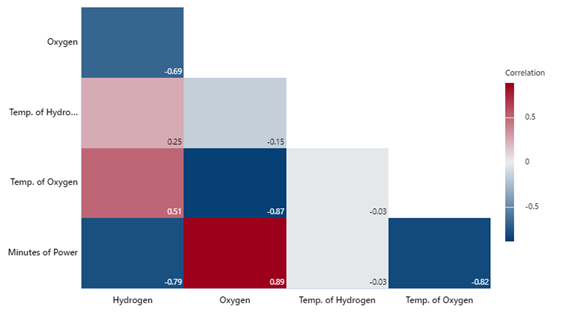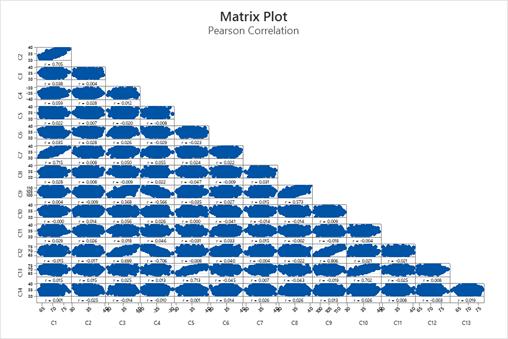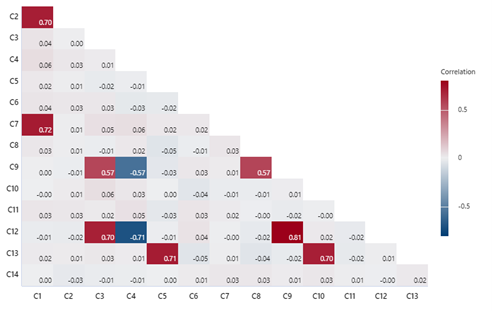With Minitab adding correlograms to its arsenal of visualizations as part of the recent Minitab Statistical Software release, I wanted to explore why these graphs are such popular and useful tools for advanced analytics.

Put simply, a correlogram – sometimes referred to as a correlation plot or a correlation matrix – is a visualization of correlation statistics. It is used to assess randomness and identify simple patterns in your data by quickly identifying variables that are strongly correlated with one another. As is the case with any data analysis, examining and understanding the structure of your data is an important first step in the predictive analytics process, and knowing whether variables are highly correlated with one another will inform your next steps.

And, as you will see, the correlogram is a fantastic visual tool to help you on your journey to better leveraging predictive analytics.

## Use a correlogram to quickly identify correlations

You may be thinking, “But I use correlation with a matrix plot to assess associations and randomness. How is this different?” Well, when you only have a few variables and a relatively small number of samples, using correlation in conjunction with a matrix plot makes a ton of sense.

But let’s take an example of an engineer who is designing fuel cells for electric cars to illustrate why a correlogram can be a powerful tool when more variables and more samples are involved.

Operating temperature is among the parameters that affect fuel cell performance, along with pressure, flow rates, and humidity, and for any fuel cell design, an optimal operating temperature must be identified.  In order to optimize the fuel cell design for performance and efficiency, the engineer needs to understand the relationship between the amount of hydrogen in the cell, the amount of oxygen in the cell, and the temperature at which the hydrogen and oxygen are pushed into the fuel cell to create energy.

The engineer plans to evaluate whether slightly higher or lower temperature chemical reactions between oxygen and hydrogen can impact the power of the fuel cell, using 14 observations for each of these measures.

After running the correlation analysis in Minitab (it is as easy as Stat-Basic Statistics-Correlation), the engineer observes the correlations among the variables in this study with both the table of correlations and a matrix plot.According to the results in the table, the Pearson correlation coefficient between hydrogen content and minutes of power is −0.791 and the p-value is 0.001. The p-value is less than the significance level of 0.05, which indicates that the correlation is significantly different from zero. The association implies that as hydrogen content increases, the minutes of power generated tends to decrease. (Recall that a correlation measures the strength of a linear association between two variables, and that is ranges between -1 [strong negative correlation] and +1 [strong positive correlation]. Correlations near zero indicate there is no strong linear association between the two variables.)

A matrix plot displays the individual associations and is a useful tool for visualizing this analysis. In the example below, note that the plot of minutes of power and hydrogen content is in the lower left corner.A matrix plot is also a useful tool for identifying potential outliers, but it is not designed for quick identification of the strongest or weakest correlations. For example, if you look at the matrix plot above, how long might it take you to decide which of these correlations is closest to either -1 or +1?

To answer that question quickly, the correlogram is a more useful tool – particularly when you are presenting this type of analysis with others who need to scan and understand the information at a glance.

Consider this same data presented is the correlogram, below (in Minitab Statistical Software: Graph-Correlogram):Did you notice how quickly your eye went to the deep red box at the bottom with the plot of minutes of power by oxygen? With correlograms, the intensity of the color is proportional to the correlation coefficient, with darker the boxes indicating stronger correlations. As a result, the correlogram provides a clear, scannable visual representation of correlations. By running the correlogram in this case, the engineer is able to understand correlations in the data with much less effort.

## Use a correlogram with larger amounts of data

Now let us consider an analysis with 14 variables and 1,000 rows of data. The specifics do not matter, this could be results from a consumer product survey or measures on a circuit board process. If you asked your team to visually pick out the strongest (close to +1 or -1) associations in the matrix plot below, how long would it take them to identify the strongest correlations?Now look at the same data presented in a correlogram, below. Notice how weak correlations are visually minimized, while your eyes are drawn to areas with high correlation. Imagine how much faster your team would identify the significant information!Understanding relationships, like correlations, between variables is a critical for robust predictive analyses. While it is easy to identify correlations when analyzing data with relatively few variables, as the number of variables and the size of datasets increase, so does the effort required to understand correlation. By harnessing the power of a correlogram, statistical analysis with Minitab becomes even better, faster, and easier – particularly for your more complex problems!